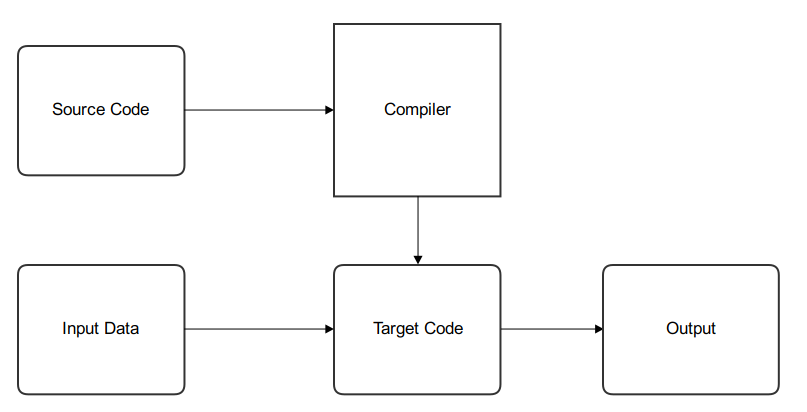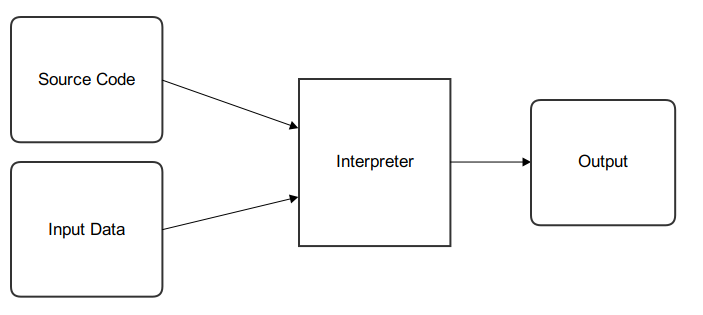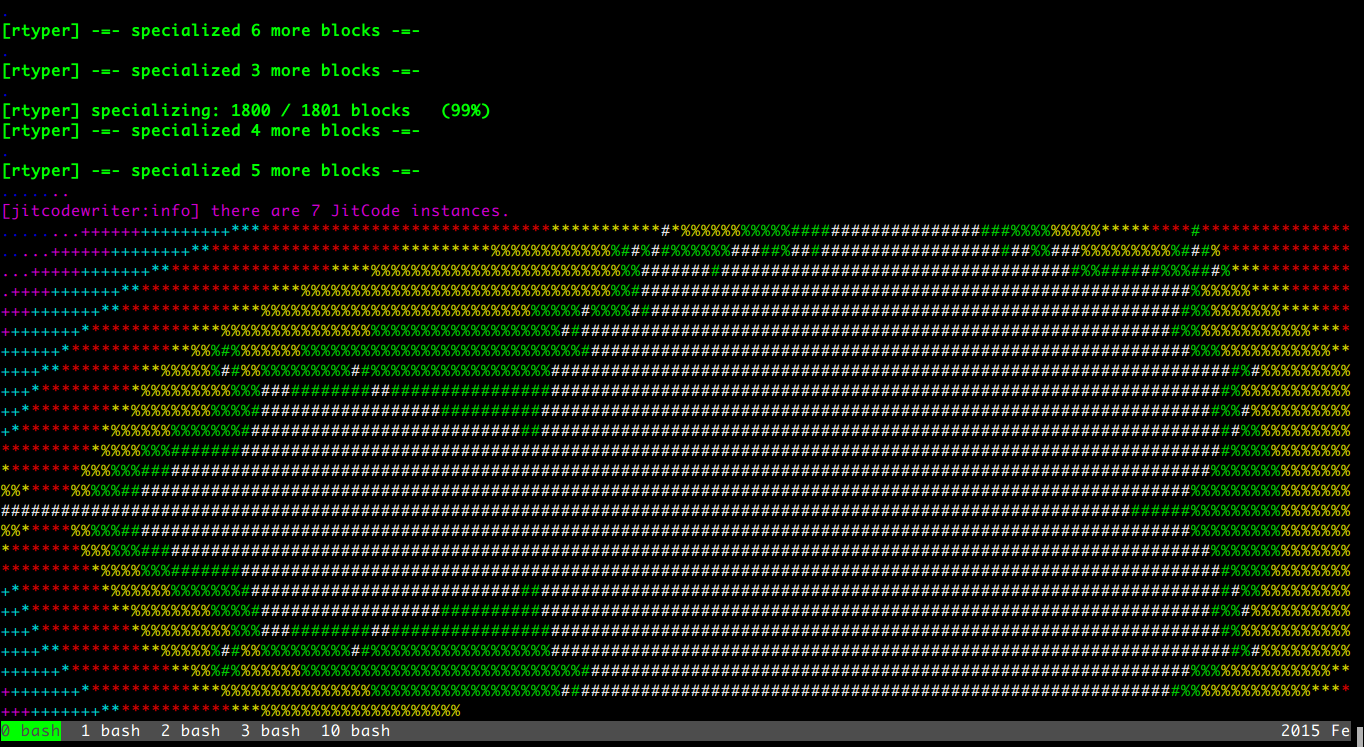# Introduction¶

Python 是一個 Dynamic Language， 官方提供了一個叫作 CPython 的 Interpreter 實作， Interpreter 讓這類 Dynamic Language 不用事先 compile 過才可以執行， 只要寫好 script 後丟進去就可以跑， 以下有 Compiler 和 Interpreter 簡陋的流程圖 :

Compiler :Interpreter :CPython 的實作只維持在很標準的方式， 相較之下並沒有花費大量的努力往更好的效能去調整， 一來應該是商業公司投資的資源量不夠， 再來應該是人力也不夠。

# Tutorial¶

PyPy 的官方 Blog 上， 在 2011 年發了兩篇由 Andrew Brown 撰寫用 PyPy 來實作 Brainfuck Interpreter 的 Tutorial， 時至今日，已經來到了 2015 年，中間也經過了許多 release， 接下來將會以 PyPy 2.5 為試驗目標， 更新一些舊 Tutorial 上需要改變的地方，並且和其他實作做初步比較。 (本練習的 code 會放在 GitHub wdv4758h/brainfuck_bench )

PyPy 這個專案其實有兩個角色

• Python 的 Interpreter 實作

• 撰寫 Dynamic Languages 的 Interpreter 的 framework

「Interpreter 的 framework」 是 PyPy 這 project 最特別的地方， 接下來這篇的重點將會放在 「撰寫 Interpreter」

• source code parser

• a bytecode interpretation loop

• lots of standard library code

## Brainfuck Interpreter - Begin¶

Brainfuck spec :

 ```> ``` 指標加一 ```< ``` 指標減一 ```+ ``` 指標指向的 byte 的值加一 ```- ``` 指標指向的 byte 的值減一 ```. ``` 輸出指標指向的 byte (ASCII) ```, ``` 輸入到指標指向的 byte (ASCII) ```[ ``` 如果指標指向的 byte 為零，向後跳到對應的 ] 指令的下一指令 ```] ``` 如果指標指向的 byte 不為零，向前跳到對應的 [ 指令的下一指令

Brainfuck to C (assuming 「ptr」 is of type 「unsigned char*」) :

brainfuck command

C equivalent

(Program Start)

```char array[infinitely large size] = {0};
char *ptr=array;
```
```>
```
```++ptr;
```
```<
```
```--ptr;
```
```+
```
```++*ptr;
```
```-
```
```--*ptr;
```
```.
```
```putchar(*ptr);
```
```,
```
```*ptr=getchar();
```
```[
```
```while (*ptr) {
```
```]
```
```}
```

```#!/usr/bin/env python
# -*- coding: utf-8 -*-

import sys

class Tape(object):
"""
因為 Brainfuck 的 code 就像是在 Tape 上操作一樣，
所以有這個 class 來處理所有 action
"""

def __init__(self):
self.thetape = 
self.position = 0

def get(self):
return self.thetape[self.position]

def set(self, val):
self.thetape[self.position] = val

def inc(self):
self.thetape[self.position] += 1

def dec(self):
self.thetape[self.position] -= 1

def advance(self):
self.position += 1
if len(self.thetape) <= self.position:
self.thetape.append(0)

def devance(self):
self.position -= 1

def main_loop(program, bracket_map):
pc = 0
tape = Tape()

while pc < len(program):

code = program[pc]

if code == '>':
tape.advance()

elif code == '<':
tape.devance()

elif code == '+':
tape.inc()

elif code == '-':
tape.dec()

elif code == '.':
# print
sys.stdout.write(chr(tape.get()))

elif code == ',':
# read from stdin
tape.set(ord(sys.stdin.read(1)))

elif code == '[' and tape.get() == 0:
# Skip forward to the matching ]
pc = bracket_map[pc]

elif code == ']' and tape.get() != 0:
# Skip back to the matching [
pc = bracket_map[pc]

pc += 1

def parse(program):
parsed = []
bracket_map = {}
leftstack = []

pc = 0
for char in program:
if char in ('[', ']', '<', '>', '+', '-', ',', '.'):
parsed.append(char)

if char == '[':
leftstack.append(pc)
elif char == ']':
left = leftstack.pop()
right = pc
bracket_map[left] = right
bracket_map[right] = left
pc += 1

return ''.join(parsed), bracket_map

def run(input_file):

with open(input_file, 'r') as f:
program, bracket_map = parse(f.read())

main_loop(program, bracket_map)

if __name__ == "__main__":
run(sys.argv)
```

### PyPy Translation¶

```def run(input_file):

with open(input_file, 'r') as f:
program, bracket_map = parse(f.read())

main_loop(program, bracket_map)

def entry_point(argv):
if len(argv) > 1:
filename = argv
else:
print("You must supply a filename")
return 1

run(filename)
return 0

def target(*args):
return entry_point

if __name__ == "__main__":
entry_point(sys.argv)
```

```import os

# sys.stdout.write(chr(tape.get()))

os.write(1, chr(tape.get()))    # 1 for stdout

# tape.set(ord(sys.stdin.read(1)))

data = os.read(0, 1)    # 0 for stdin, 1 for one byte
if data != '':
tape.set(ord(data))
```

```hg clone https://bitbucket.org/pypy/pypy
```

```pypy/rpython/bin/rpython example2.py
```

 File Size 290552 bytes Translation Time 56.5 s

Test File

Execution Time

mandel.b

68.61 s

```./example2-c mandel.b
```

Bash 裡有自己的 time command 可以看執行時間， 但是如果要更多資訊的話 (-v)，需要 GNU 版的 time command

```sudo pacman -S time
```
```time -v ./example2-c mandel.b
```

• 符合 RPython 語法、功能

• `target` 這個 function 回傳進入的 function

### More Jobs¶

(針對 C backend compile 時的 `-j` 參數)

### Garbage Collection¶

• boehm

• ref (default)

• semispace

• statistics

• generation

• hybrid

• minimark

• incminimark

• none

## Brainfuck Interpreter - JIT¶

• 簡單 (基於原本的 Interpreter 上，只要做少許修改就能有 JIT)

• Maintainable (不會因為加了 JIT 就造成需要開另外的 project 分別 maintain)

• 夠快 (雖然 JIT 是生出來的，但是也要速度也要夠快)

(詳細訊息請看 RPython Documentation - JIT )

```from rpython.rlib.jit import JitDriver
jitdriver = JitDriver(greens=['pc', 'program', 'bracket_map'], reds=['tape'])
```

```jitdriver.jit_merge_point(pc=pc, tape=tape, program=program, bracket_map=bracket_map)
```

```pypy/rpython/bin/rpython --opt=jit example3.py
```

• import `JitDriver` 進來，把 green 和 red 變數分好

• 在 main loop 裡 while 一開始的地方 call `jit_merge_point` 把變數傳進去

• translate 的時候加上 `--opt=jit` 參數

 File Size 5954320 bytes Translation Time 977.4 s

Test File

Execution Time

mandel.b

27.64 s```def jitpolicy(driver):
from rpython.jit.codewriter.policy import JitPolicy
return JitPolicy()
```

## Tracing JIT¶

Interpreter 執行的是我們寫的 interpreter code， 當發現 target laugange (Brainfuck) 寫的某段 code 很常跑時， 會把這部份標成 「Hot」，並且會做追蹤，當下一次進到這個循環的時候， interpreter 會進入 tracing mode，把每個指令紀錄下來，循環結束後， tracing mode 就停止，把追蹤紀錄丟給 optimizer， 接著丟給 assembler，產生 machine code 在之後執行時使用。

### Debug and Trace Log¶

```def get_location(pc, program, bracket_map):
return "%s_%s_%s" % (
program[:pc], program[pc], program[pc+1:]
)

jitdriver = JitDriver(
greens=['pc', 'program', 'bracket_map'],
reds=['tape'],
get_printable_location=get_location
)
```

```pypy/rpython/bin/rpython --opt=jit example4.py
```

```PYPYLOG=jit-log-opt:logfile ./example4-c test.b
```

```[3c091099e7a4a7] {jit-log-opt-loop
```

```[3c091099eae17d] jit-log-opt-loop}
```

## Optimize¶

### elidable (old : purefunction)¶

```from rpython.rlib.jit import elidable

@elidable
def get_matching_bracket(bracket_map, pc):
return bracket_map[pc]

# 下面把查 bracket_map 的地方換掉
```

 File Size 5852352 bytes Translation Time 960.2 s

Test File

Execution Time

mandel.b

9.58 s

### Delay Output¶

```class Tape(object):
def __init__(self):
self.thetape = 
self.position = 0
self.output = ''
self.output_threshold = 50

def get(self):
return self.thetape[self.position]

def set(self, val):
self.thetape[self.position] = val

def inc(self):
self.thetape[self.position] += 1

def dec(self):
self.thetape[self.position] -= 1

def advance(self):
self.position += 1
if len(self.thetape) <= self.position:
self.thetape.append(0)

def devance(self):
self.position -= 1

def clear(self):
if self.output:
os.write(1, self.output)    # 1 for stdout
self.output = ''

def read(self):
self.clear()

data = os.read(0, 1)    # 0 for stdin, 1 for one byte

if data:
self.set(ord(data))

def write(self):
self.output += chr(self.get())

if len(self.output) > self.output_threshold:
os.write(1, self.output)    # 1 for stdout
self.output = ''
```

### Zero¶

Brainfuck code 裡面的 「[-]」 這樣的 loop 其實就是把目前指到的值歸零， 所以可以直接把它 assign 成零，不要再慢慢減了， 這樣換掉後可以有些許的提升

### Compact¶

Brainfuck 的 code 裡面常常會出現連續的 「+」 或 「-」 或 「<」 或 「>」， 但是這是可以一次完成的 (連續的 「>」、」<」 都可以和起來，」+」、」-」 也可以)， 不需要一個一個慢慢加、一個一個慢慢移， 所以如果把這部份處理掉， 做更有效率的計算， 可以獲得一部份的效能提升 ~

## 總結¶

```What blocks Ruby, Python to get Javascript V8 speed?

Nothing.

Well, okay: money. (And time, people, resources, but if you have money, you can buy those.)
```

1. 用 RPython 寫你的 Interpreter (需要有 target function)

2. 針對 main loop 把變數分類、call JIT 的 Driver class

3. 丟下去 toolchain 轉換

4. 效能還不夠時，找出不會變得地方用 「elidable」 decorator 做告知

# 額外紀錄¶

## RPython’s print statement¶

RPython 裡面其實是有支援 print statement 的， 但是那大多只用於 debug， 多數情況都用 os.read / os.write， 不過其實可以用類似 「os.fdopen(1)」 的方法來拿到 stdout (不過要在 RPython function 裡，而不是 module global)， 所以其實可以做到當我在 RPython 用 sys.stdout 時其實後面是 call rfile.py 裡面類似 getstdout() 的 function， 它會取得並且 cache 用 os.fdopen() 取得的 rfile。

## Comple with Clang¶

```I guess clang produces subtly different assembler that throws off "trackgcroot"
you can use --gcrootfinder=shadowstack
that will be slightly slower
(~10% and only before jit-compilation)
(trackgcroot is a hack used with --gcrootfinder=asmgcc, which is enabled only on Linux;
usually we have to fix it slightly for every new version of gcc...)
```

## string replace¶

RPython 裡的 str 目前只支援 char 的 replace， 但是可以從 `rpython/rlib/rstring.py` 裡找到 replace function 來替代， `from rpython.rlib.rstring import replace``replace(string, old, new, max)`

```   wdv| any reason that RPython's string replace only works for char args ?
ronan| wdv: no very good reasons
ronan| wdv: it would be a bit of work to implement and interpreters are usually better off writing their own
replace at a low level
cfbolz| ronan, wdv: there is even a usable implementation, in rlib.rstring, I think
```

## Embedding PyPy¶

```mstuchli| This is prolly a stupid question, but the libpypy-c.so is a new thing, correct? What's it for?
fijal| mstuchli: for embedding
arigato| mstuchli: for embedding pypy into some other program, more precisely; now the "pypy" executable is
very small and just calls libpypy-c.so, but other programs may call it too
```